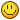# Getting positions on your screen

## Recommended Posts

Hi,

I'd like to share my way of getting a Position on your screen to make something like this:The cool thing is these lines rotate with your camera.

```    public static Point getPositionOnScreen(Script script, Position middle /* myPosition = default */, Position targetPosition) {
final Point drawAt = new Point(642, 83); // Default miniMap centre 642, 83
final double zoom = 0.25; // Default: 0.25

// Getting a direction.
int x = middle.getX() - targetPosition.getX();
int y = middle.getY() - targetPosition.getY();

// Getting the correct angle.
double angle = (Math.toDegrees(Math.atan2(y, x)) - script.client.getCameraYawAngle() - 90) * Math.PI / 180;

// Getting the position on the screen.
x = (int) StrictMath.round(StrictMath.sin(angle) * (middle.distance(targetPosition) / zoom));
y = (int) StrictMath.round(StrictMath.cos(angle) * (middle.distance(targetPosition) / zoom));

return new Point(drawAt.x + x, drawAt.y + y);
}
```

Credits would be kind.

Enjoy.

Edited by jelknab
•3

##### Share on other sites

What are the uses of this? Its really cool##### Share on other sites

Thanks! Very useful!